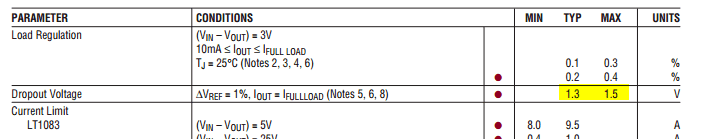# Adjustable Voltage Regulator Output Voltage Range

## Homework Statement

FIGURE 2 shows an adjustable voltage regulator using the LT1083

.The LT1083 develops a 1.25V reference voltage between the output and the
adjust terminal. By placing a resistor R1 between these two terminals, a constant
current is caused to flow through R1 and down through R2 to set the overall
output voltage.
If VIN=18V, determine the range over which the output voltage can be varied.

## Homework Equations

$V OUT=V REF*(1+ \frac{R2}{R1})$

## The Attempt at a Solution

$V OUT=1.25*(1+ \frac{1000}{90.9})=15V$

$V OUT=1.25*(1+ \frac{0}{90.9})=1.25V$

The output voltage range being 1.25-15V

This is the only equation I can find relating to the output voltage of the regulator. By making two calulations using 0 and 1K ohms of R2 this would give me the range I am looking for, but what is confusing me is the fact that they have given me a V IN. Is this just a false trail or am I actually missing something?

#### Attachments

•image1.JPG
79.1 KB · Views: 658
•Enochfoul

CWatters
Homework Helper
Gold Member
Hint...Read the problem statement carefully then work out the voltage drop across each resistor. Mark it on the circuit and see what that tells you about vout.

Hint...Read the problem statement carefully then work out the voltage drop across each resistor. Mark it on the circuit and see what that tells you about vout.
If I ADJ is 10mA then the volt drop across R1 is 0.909V and R2 is 10V. I'm struggling to see where this leads?

*Sorry this is totally wrong. I'm don't see what current would be going through R1 and R2....

gneill
Mentor

## The Attempt at a Solution

$V OUT=1.25*(1+ \frac{1000}{90.9})=15V$

$V OUT=1.25*(1+ \frac{0}{90.9})=1.25V$

The output voltage range being 1.25-15V

This is the only equation I can find relating to the output voltage of the regulator. By making two calulations using 0 and 1K ohms of R2 this would give me the range I am looking for, but what is confusing me is the fact that they have given me a V IN. Is this just a false trail or am I actually missing something?
Your results look good. The only criteria that would make Vin important would be the device's specification for the minimum Vin-Vout for proper operation; the device needs some potential difference leeway to work with between the supply voltage and the regulated output voltage. It can't produce 10 V from a 5 V source, for example. This is called the dropout voltage for the device.

Check the device datasheet to find out what this value is and hence whether it comes into play over the range of the given setup.

Your results look good. The only criteria that would make Vin important would be the device's specification for the minimum Vin-Vout for proper operation; the device needs some potential difference leeway to work with between the supply voltage and the regulated output voltage. It can't produce 10 V from a 5 V source, for example. This is called the dropout voltage for the device.

Check the device datasheet to find out what this value is and hence whether it comes into play over the range of the given setup.
When you say minimum Vin-Vout, the LT1083 datasheet has many Vin-Vout's. Which parameter should I be looking at on the datasheet for this?

I presume it would be line regulation which is 1.5<=(Vin-Vout)<=15, but this puts my answers outside of that range?

gneill
Mentor
Look for the Dropout Voltage.

gneill
Mentor
I've uploaded an image of the data sheet. The dropout voltage just states, "change in V REF=1%".
Those are the measurement conditions for the parameter. What you want to look at are any MIN, TYP, and MAX values for it:So it looks like you need to maintain, worst case, a 1.5 V buffer between Vin and Vout.

Those are the measurement conditions for the parameter. What you want to look at are any MIN, TYP, and MAX values for it:
View attachment 98328
So it looks like you need to maintain, worst case, a 1.5 V buffer between Vin and Vout.
Ah okay so the highest voltage of 15V still works just fine.
Many thanks!

CWatters
Homework Helper
Gold Member
I'm don't see what current would be going through R1 and R2....

The problem statement tries to explain how it works but isn't very clear. It says..

The LT1083 develops a 1.25V reference voltage between the output and the
adjust terminal. By placing a resistor R1 between these two terminals, a constant current is caused to flow through R1 and down through R2

What's happening here is that the LT1083 maintains a voltage of 1.25V across R1. This means the current through R1 must be 1.25/90.9 = 13.75mA

If you assume no current goes into or out of the Adjust Pin then the current in R2 is the same as R1 = 13.75mA.

So now you can work out the voltage across R1 and R2.

R1 and R2 are connected between 0V and Vout.

PS: If you use this method you get the same answer as using the equation you found, except this way helps you understand how the regulator works.